## 26.0 g of copper pellets are removed from a 300∘C oven and immediately dropped into 120 mL of water at 21.0 ∘C in an insulated cup.What will

Question

26.0 g of copper pellets are removed from a 300∘C oven and immediately dropped into 120 mL of water at 21.0 ∘C in an insulated cup.What will the new water temperature be?

in progress 0
6 months 2021-07-28T04:55:00+00:00 1 Answers 12 views 0

The new water temperature is 26.4 °C

Explanation:

Given;

mass of copper,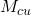= 26 g = 0.026 kg

temperature of copper, t = 300 °C

volume of water, V = 120 mL = 0.12 L

temperature of water, t = 21 °C

density of water, ρ = 1 kg/L

mass of water = density x volume

mass of water = (1 kg/L) x 0.12 L = 0.12 kg

heat lost by copper = heat gained by water

Both copper and water reach final temperature, T

Heat gained by water,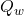=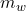cΔθ =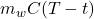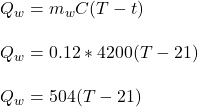Heat lost by copper is given by;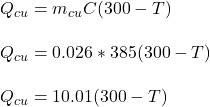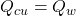504(T- 21) = 10.01(300 – T)

504 T – 10584 = 3003 – 10.01 T

504 T + 10.01 T= 3003 + 10584

514.01 T = 13587

T = (13587) / 514.01

T = 26.4 °C

Therefore, the new water temperature is 26.4 °C